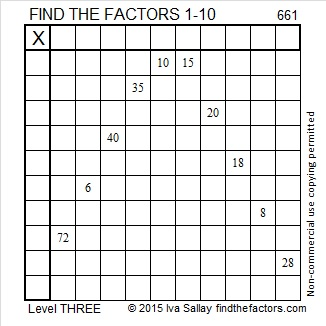# 1528 Candy Corn

### Today’s Puzzle:

To solve this Level 3 Candy Corn Halloween puzzle, first, find the factors that will work with the clues in the top and bottom rows. Then work you way down row by row filling in factors as you go.

When you get to the 8 in this puzzle, will the factors be 8 × 1 or 4 × 2? Two of those factors will be eliminated because they already appear in the first column. The other two remain possibilities, but one of those factors cannot appear in any other place in that first column, so that is the one you will want to choose. Have a sweet time solving this puzzle!Here is a plain version of the same puzzle:### Factors of 1528:

1528 is divisible by two because it is even.

1528 is divisible by four because its last two digits (in the same order) make a number, 28, which is divisible by 4.

Can 1528 be evenly divided by 8? Yes. Here’s a quick way to know: 28 is divisible by 4, but not by 8, AND 5 is odd, so 1528 is divisible by 8, as is every other number ending in 528.

• 1528 is a composite number.
• Prime factorization: 1528 = 2 × 2 × 2 × 191, which can be written 1528 = 2³ × 191.
• 1528 has at least one exponent greater than 1 in its prime factorization so √1528 can be simplified. Taking the factor pair from the factor pair table below with the largest square number factor, we get √1528 = (√4)(√382) = 2√382.
• The exponents in the prime factorization are 3 and 1. Adding one to each exponent and multiplying we get (3 + 1)(1 + 1) = 4 × 2 = 8. Therefore 1528 has exactly 8 factors.
• The factors of 1528 are outlined with their factor pair partners in the graphic below.### Another Fact about the Number 1528:

Since 1528 is divisible by 8 but not by 16, it can be written as the sum of 16 consecutive numbers:
88+89+90+91+92+93+94+95+96+97+98+99+100+101+102+103=1528.

Note that 95 + 96 = 191, and 8 × 191 = 1528.
Likewise, 94 + 97 = 1528,
93 + 98 = 1528, and so forth until we get to…
88 + 103 = 1528.

# 927 Candy Corn

Candy corn is a traditional Halloween candy.

Figure out what number goes in the top cell of the first column of this level three candy corn puzzle, and work your way down the first column, cell by cell, to make this puzzle a treat to complete.Print the puzzles or type the solution on this excel file: 12 factors 923-931

Fibonacci numbers begin with 1, 1, with the rest of the numbers in the sequence being the sum of the previous two.

Tribonacci numbers begin with 0, 0, 1 with the rest of the numbers in the sequence being the sum of the previous THREE.

The first 15 tribonacci numbers are 0, 0, 1, 1, 2, 4, 7, 13, 24, 44, 81, 149, 274, 504, 927. Thank you, OEIS.org for that fun fact.

• 927 is a composite number.
• Prime factorization: 927 = 3 × 3 × 103, which can be written 927 = 3² × 103
• The exponents in the prime factorization are 2 and 1. Adding one to each and multiplying we get (2 + 1)(1 + 1) = 3 × 2  = 6. Therefore 927 has exactly 6 factors.
• Factors of 927: 1, 3, 9, 103, 309, 927
• Factor pairs: 927 = 1 × 927, 3 × 309, or 9 × 103
• Taking the factor pair with the largest square number factor, we get √927 = (√9)(√103) = 3√103 ≈ 30.44667

# 661 Candy Corn

25² + 6² = 661

661 is the hypotenuse of the primitive Pythagorean triple 300-589-661 which was calculated using 2(25)(6), 25² – 6², 25² + 6².

661 is also the sum of the three prime numbers from 211 to 227. What is the prime number in the middle of the sum?

This Find the Factors puzzle is supposed to look like a piece of candy corn. 🙂Print the puzzles or type the solution on this excel file: 10 Factors 2015-10-26

Here’s the puzzle without the possibly distracting color:————————————

• 661 is a prime number. 659 and 661 are twin primes.
• Prime factorization: 661 is prime.
• The exponent of prime number 661 is 1. Adding 1 to that exponent we get (1 + 1) = 2. Therefore 661 has exactly 2 factors.
• Factors of 661: 1, 661
• Factor pairs: 661 = 1 x 661
• 661 has no square factors that allow its square root to be simplified. √661 ≈ 25.70992.How do we know that 661 is a prime number? If 661 were not a prime number, then it would be divisible by at least one prime number less than or equal to √661 ≈ 25.7. Since 661 cannot be divided evenly by 2, 3, 5, 7, 11, 13, 17, 19, or 23, we know that 661 is a prime number.

Here’s another way we know that 661 is a prime number: Since 25² + 6² = 661, and 25 and 6 have no common prime factors, 661 will be prime unless it is divisible by a primitive Pythagorean hypotenuse less than or equal to √661 ≈ 25.7. Since 661 is not divisible by 5, 13, or 17, we know that 661 is a prime number.

—————————————————————————————————

A Logical Approach to solve a FIND THE FACTORS puzzle: Find the column or row with two clues and find their common factor. (None of the factors are greater than 10.)  Write the corresponding factors in the factor column (1st column) and factor row (top row).  Because this is a level three puzzle, you have now written a factor at the top of the factor column. Continue to work from the top of the factor column to the bottom, finding factors and filling in the factor column and the factor row one cell at a time as you go.